Question

Vectors

A vector field V(r) is given in cylindrical coordinates by

\mathbf{V}(\mathbf{r})=\rho^{2} \hat{\boldsymbol{\rho}}+\frac{z^{2}}{\rho} \cos \phi \hat{\boldsymbol{\phi}}+2 z \sin \phi \hat{\mathbf{z}}

Calculate V . V and ▼ × V.

Determine if the vector field V is (i) solenoidal, (ii) irrotational (iii) conservative in the whole space and explain your answer.

) A path C starts from the point W = (1,0, 0), to the point X = (1,0, 1) along thestraight line x = 1, y = 0 and z from 0 to 1, then from X to Y = (-1,0, 1) alongthe semicircle with z = 1, p= 1 and ø ranging from 0 to 7 anticlockwise, then tothe point Z = (-1,0,0) along the straight line x = -1, y = 0 and z from 1 to0, and finally from Z to W along the semicircle with p= 1, z = 0 and ø from Ato 0 clockwise. Draw the path C in Cartesian coordinates and explicitly calculatethe line integral

I_{C}=\oint_{C} \mathbf{v} \cdot d \mathbf{r}

Calculate the surface integral

I_{S}=\int_{S} \nabla \times \mathbf{V} \cdot d \mathbf{S}

where S is the surface on the curved part of the cylinder enclosed by the loop C.Verify with your explicit calculations of Ic and Is the Stokes' theorem given by

\int_{S}(\boldsymbol{\nabla} \times \mathbf{V}) \cdot d \mathbf{S}=\oint_{C} \mathbf{V} \cdot d \mathbf{r}

\text { Find the function } f(\rho, \phi, z) \text { such that } \mathbf{V}=\boldsymbol{\nabla} f \text { with } f(0,0,0)=0 \text {. }Verified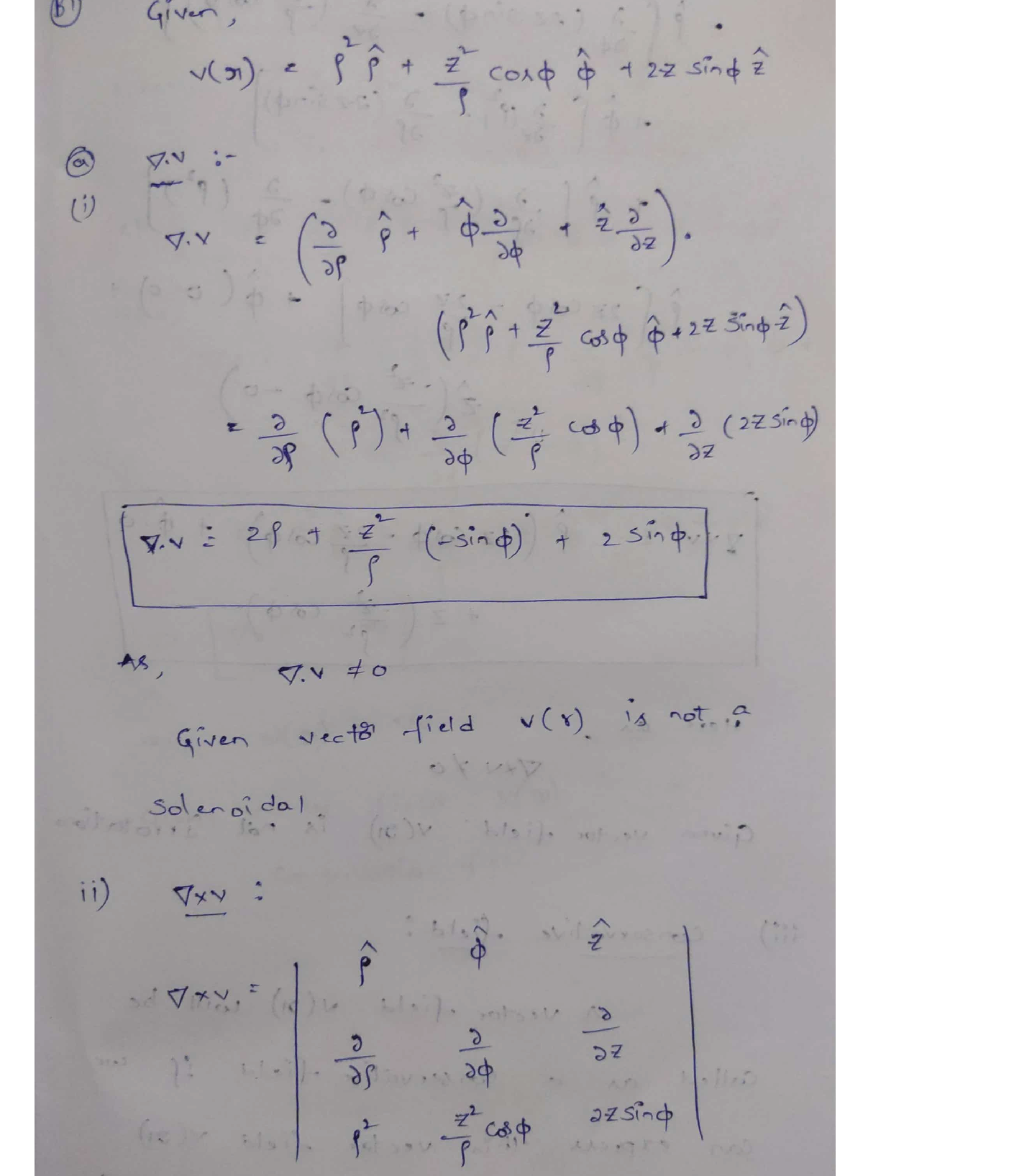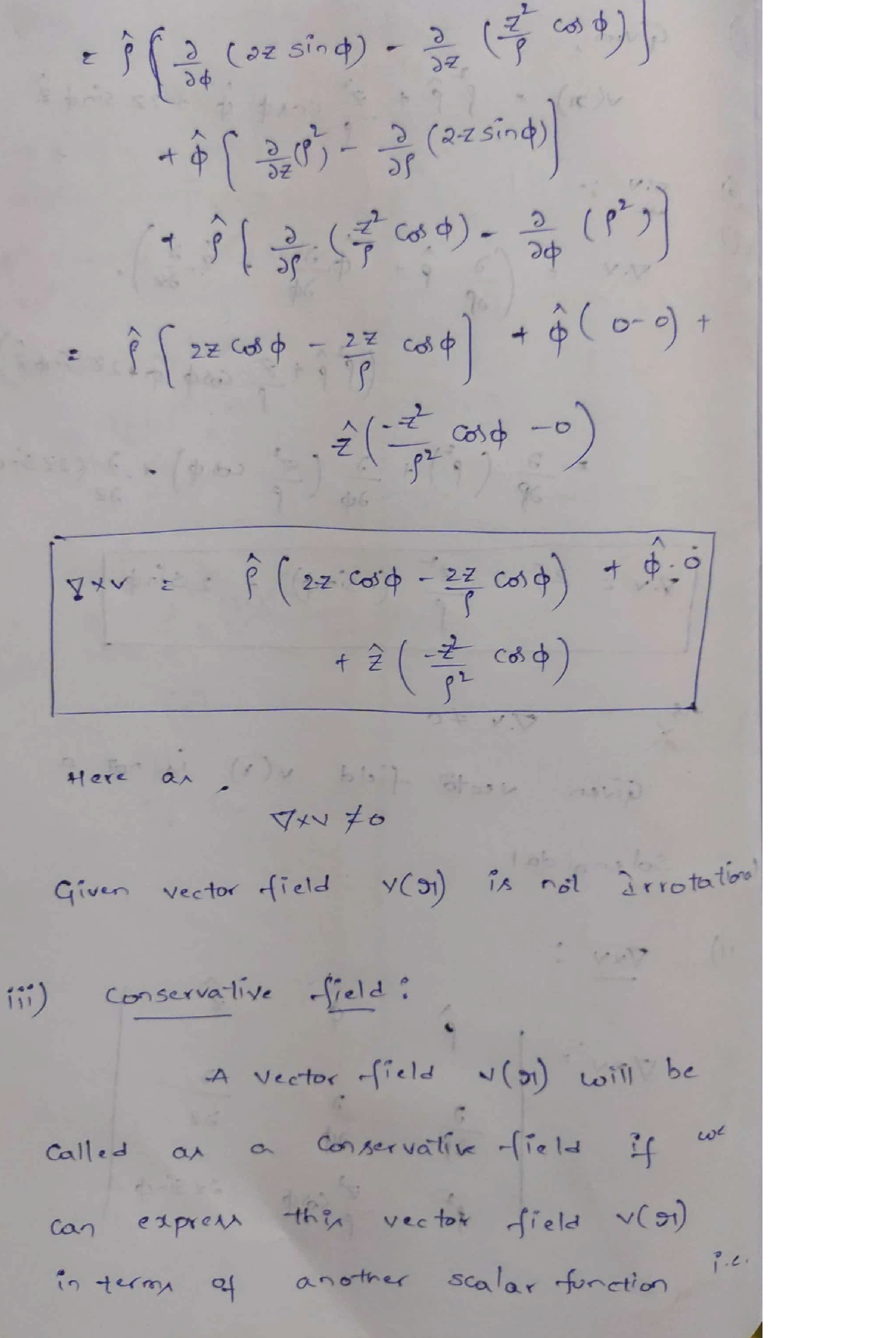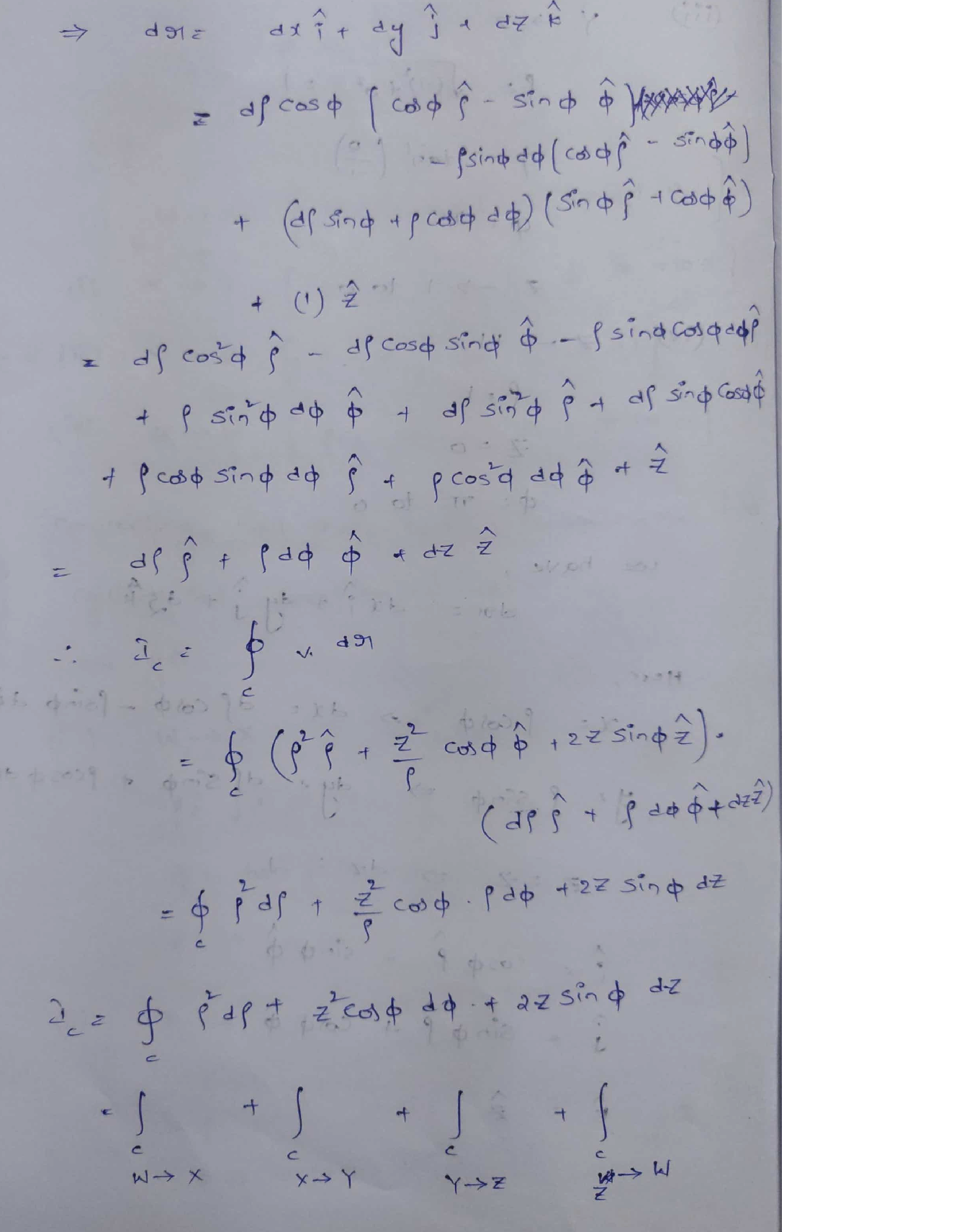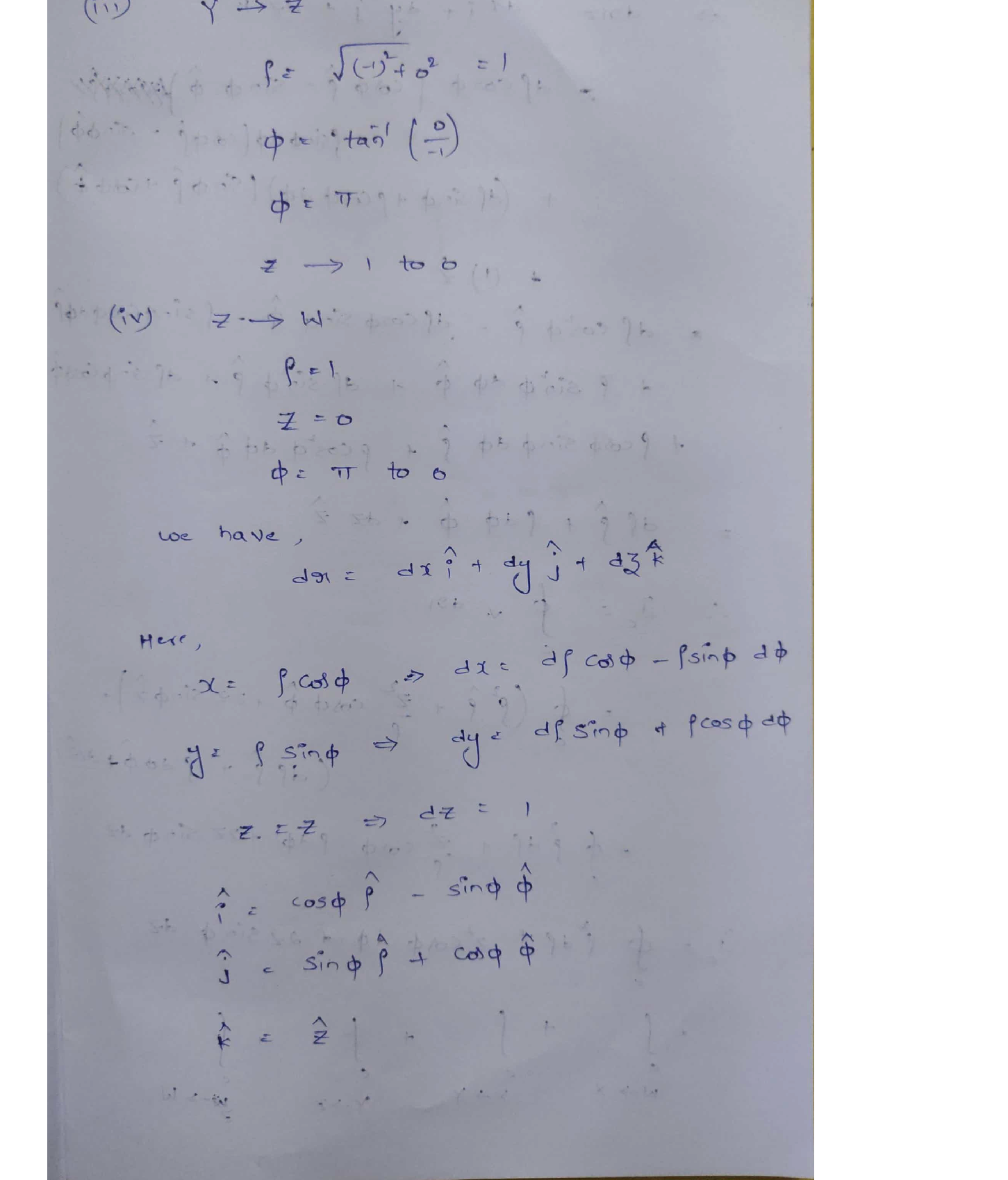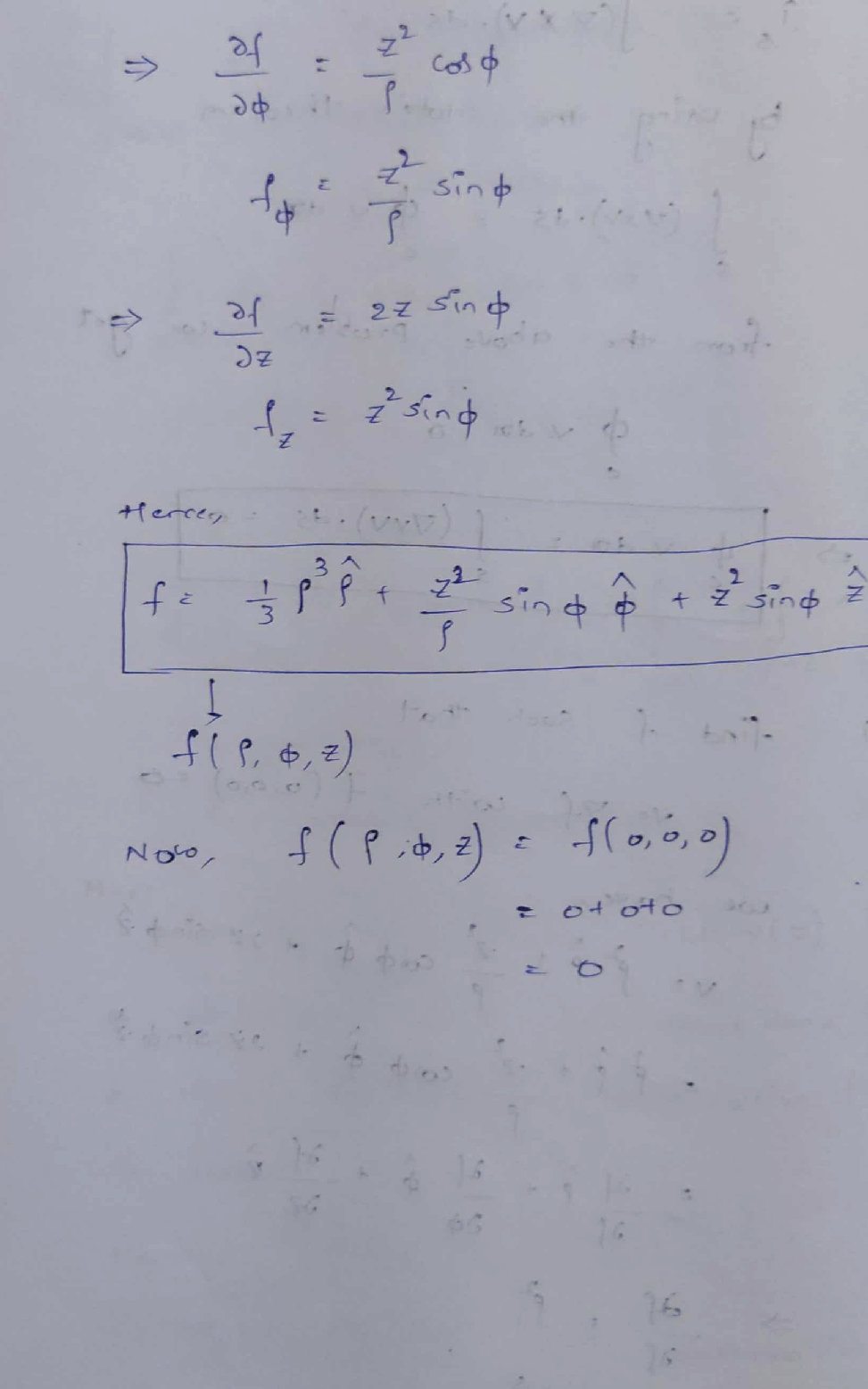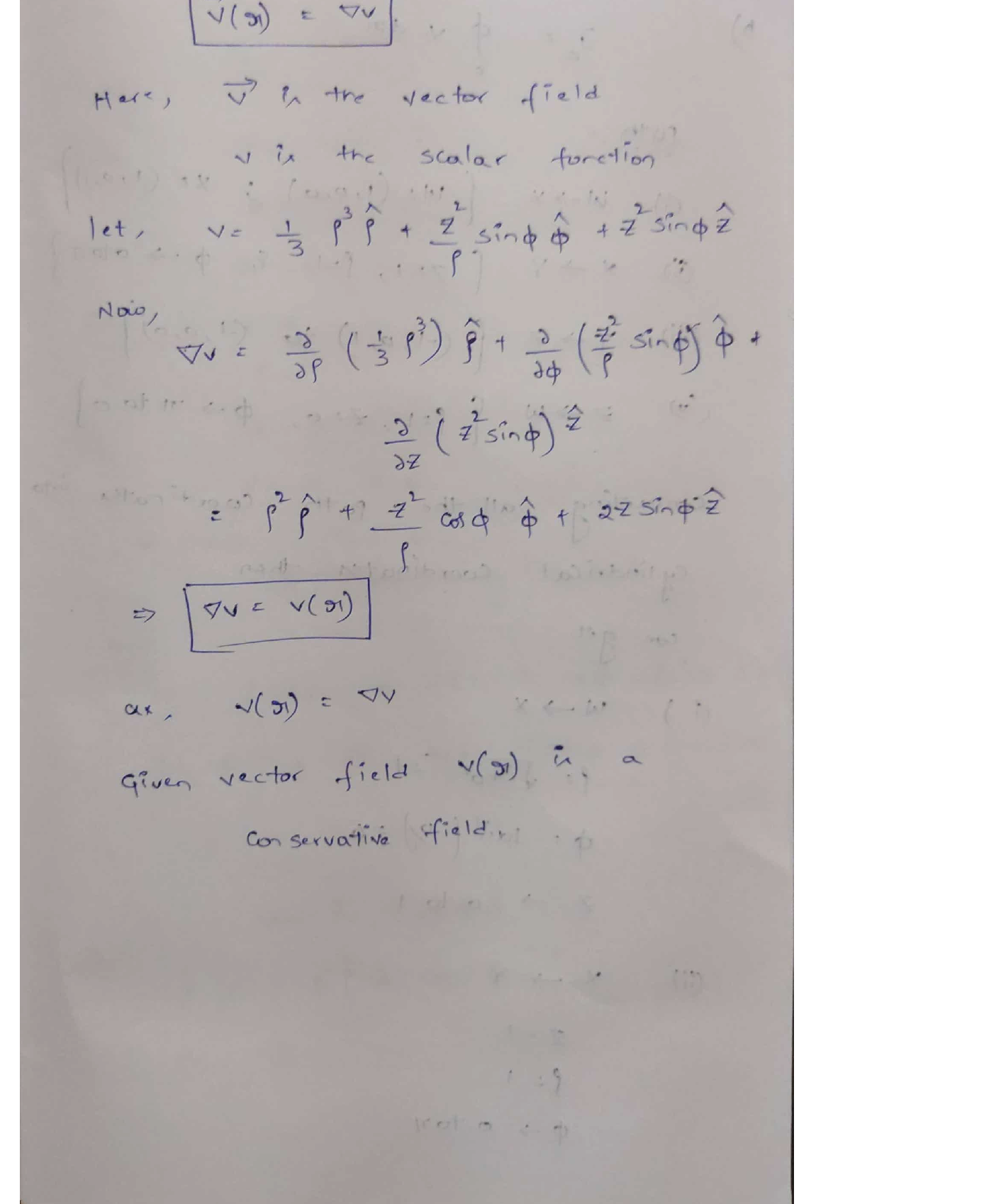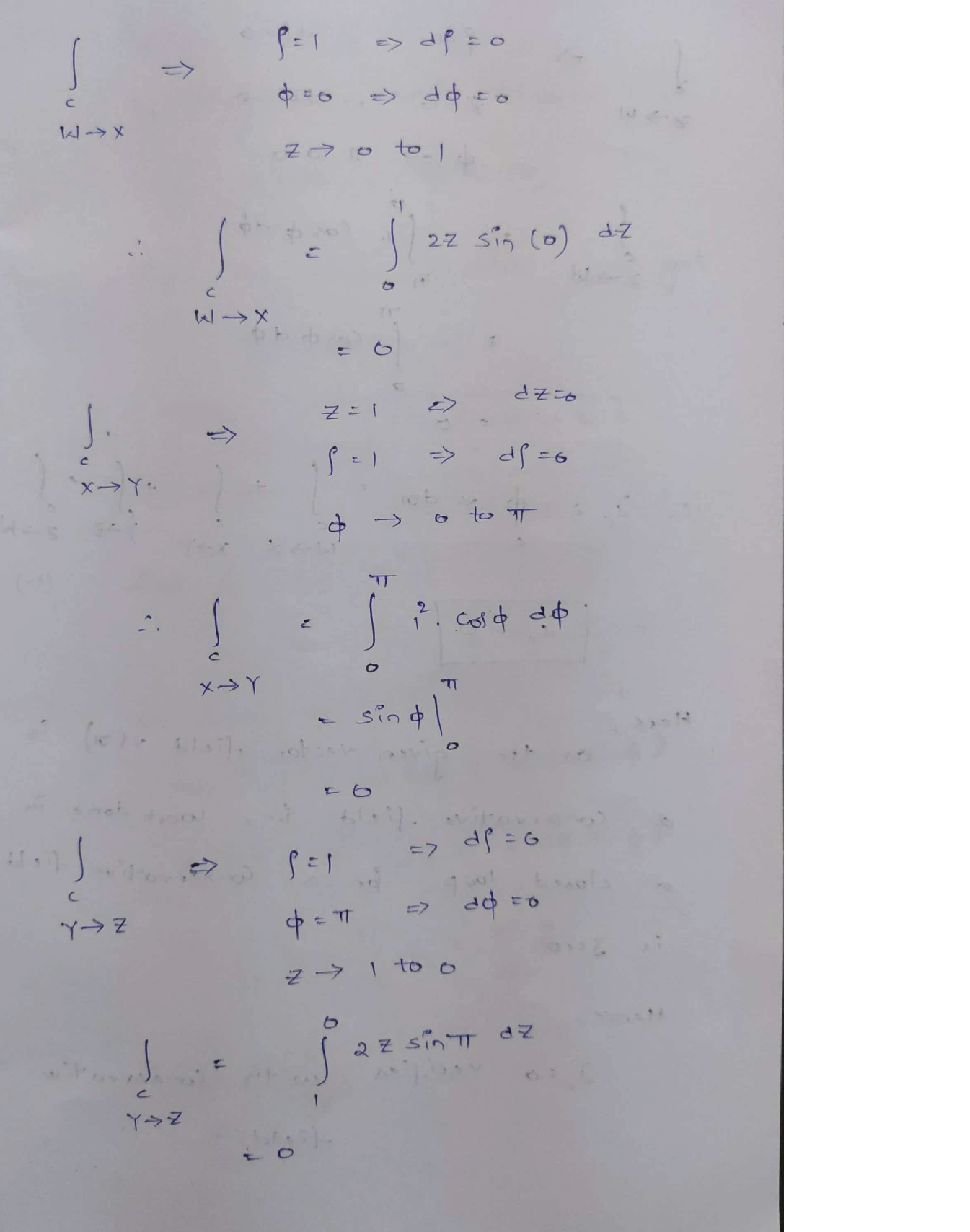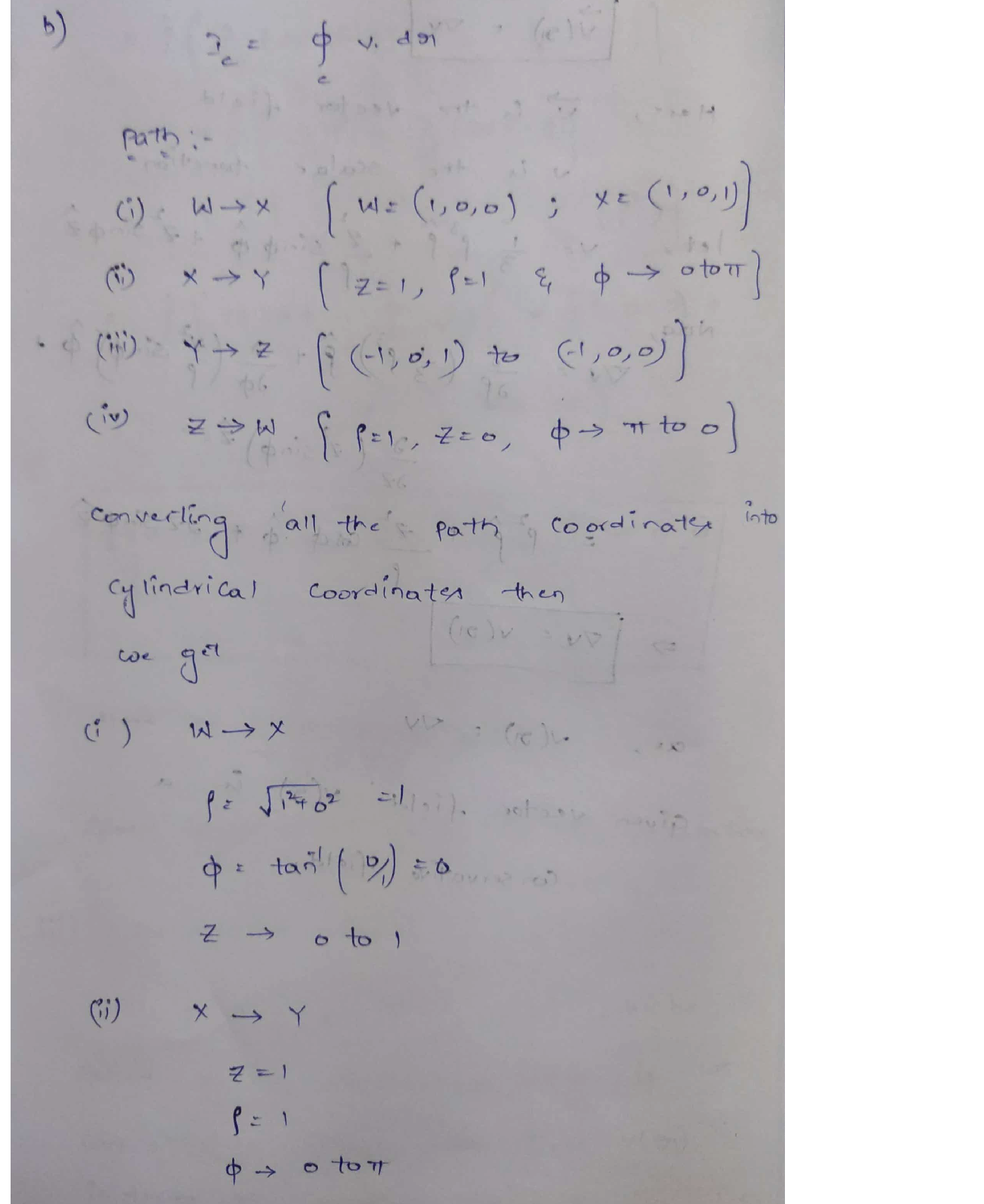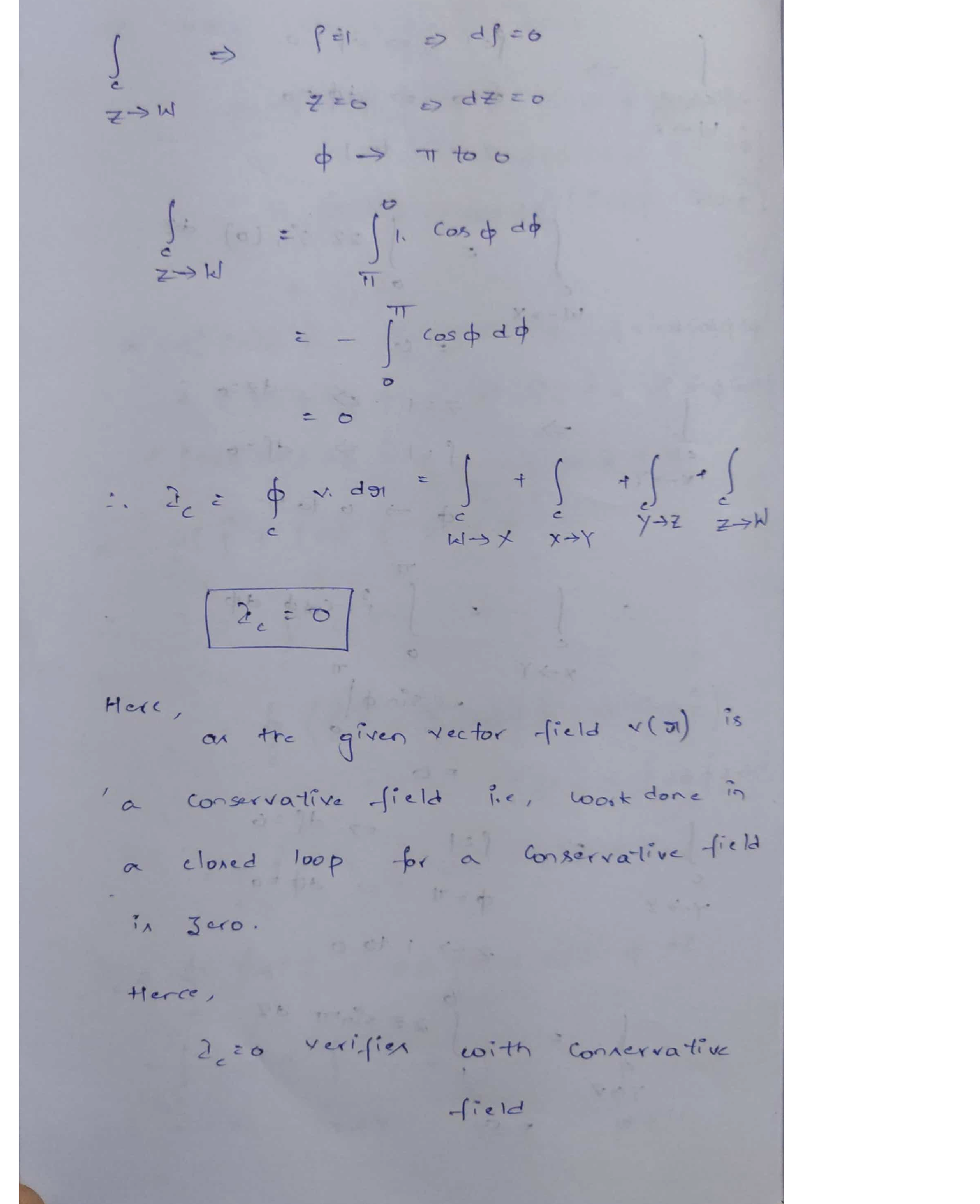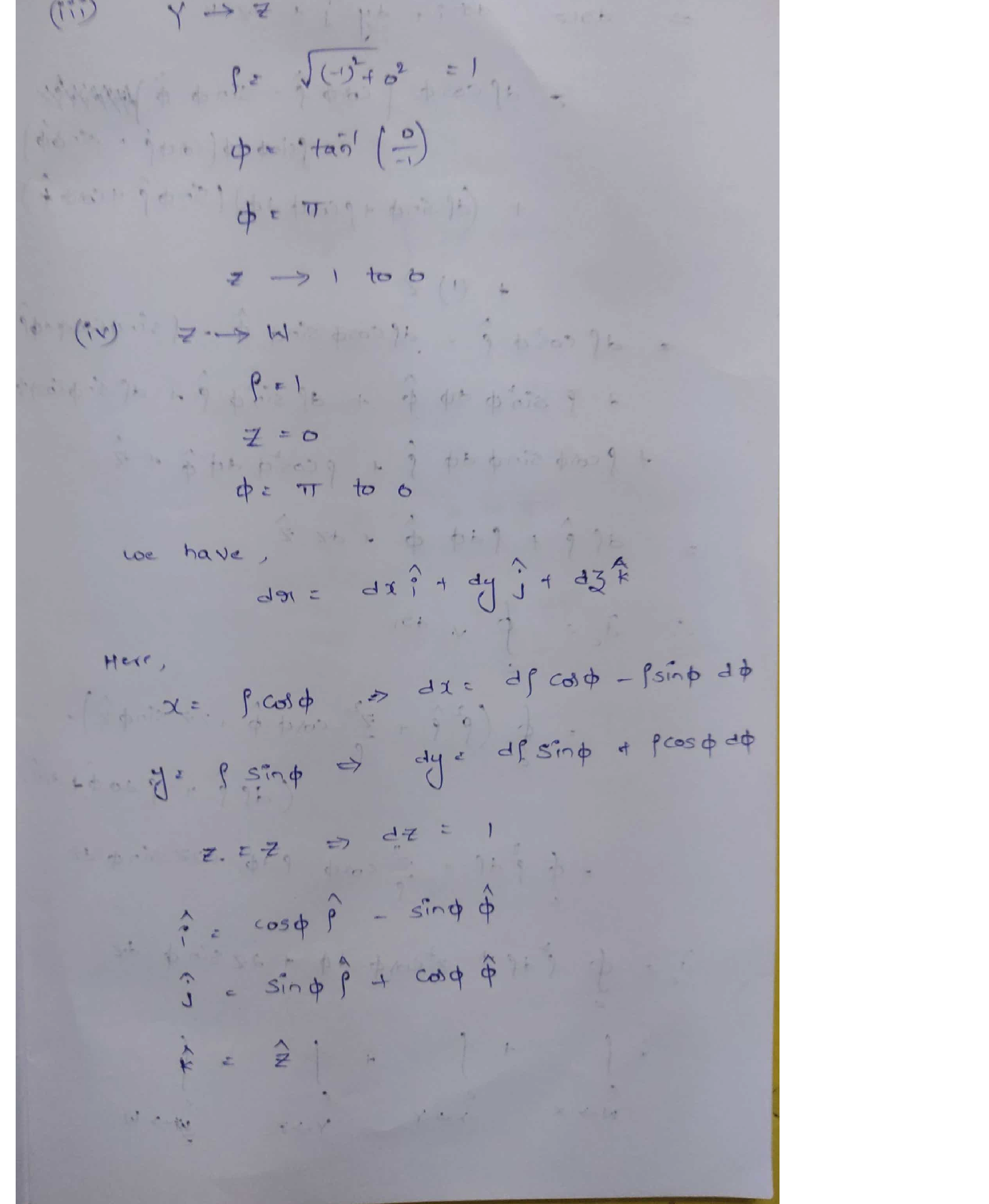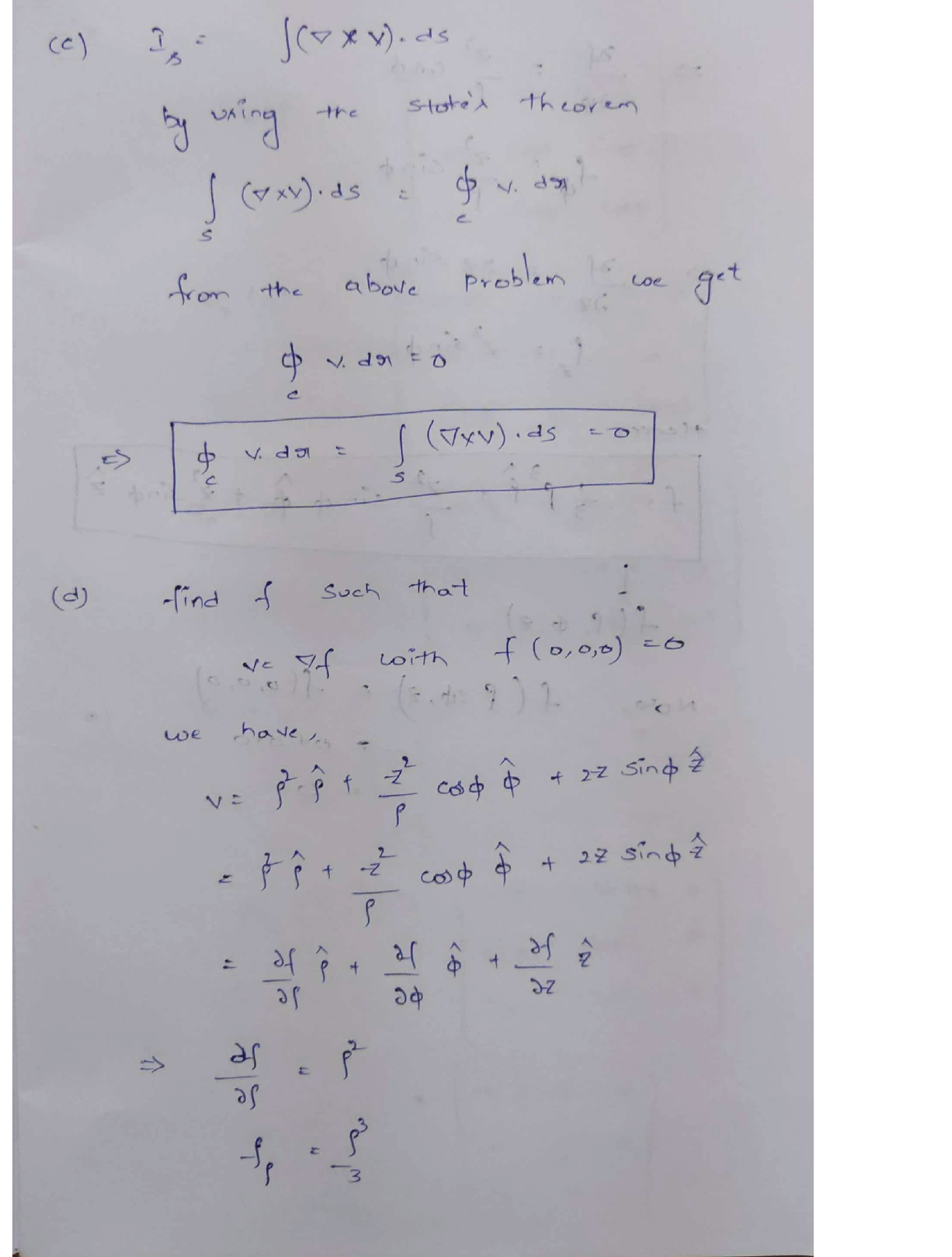### Question 45499Vectors

Suppose that
\mathbf{A}=\left(\begin{array}{cccc} 1 & x & y & 1 \\ 1 & x & x & x \\ x & 1 & x y & y \\ x & x & x y & 1 \end{array}\right) \in \mathscr{M}_{4,4}(\mathbb{R})
Show that A is not invertible if and only if either x = y, x =1 or xy = 1.
Let V and W be 3-dimensional vector spaces over R, Bị = {V1, V2, V3} be an ordered basis for V and B2 = {w1,w2, w3} be an ordered basis for W. Define a linear transformation T :V → W by
T\left(\mathbf{v}_{1}\right)=\mathbf{w}_{1}+\mathbf{w}_{2}
T\left(\mathbf{v}_{2}\right)=\mathbf{w}_{1}-\mathbf{w}_{2}+\mathbf{w}_{3}
T\left(\mathbf{v}_{3}\right)=\mathbf{w}_{3}
(i) Write down the matrix A representing T with respect to the ordered bases B1 and
(ii) Calculate the dimension of the kernel of T and of the image of T.
(iii) Show that B3 = {v1,V1 + V2, V1 + V2 + V3 } is a basis for V and B4 = {w1 -
W2, W2 +W3, W1 – W3} is a basis for W.
) Find the matrix which represents T with respect to the ordered bases B3 and B4.

### Question 45483Vectors

Prove that
\delta\left[a\left(x-x_{1}\right)\right]=\frac{1}{a} \delta\left(x-x_{1}\right) .
\text { Note. If } \delta\left[a\left(x-x_{1}\right)\right] \text { is considered even, relative to } x_{1} \text {, the relation holds for negative } a
and 1/a may be replaced by 1/la|.

### Question 41474Vectors

Problem 6. (Exercise 6 in the lecture on6.23) Finish the proof of either of the following statements:
(a) Let S =then S contains a subset S', such that S' is a basis ofV.{v1, v2, ..., Um} be a spanning set of a finite dimensional F-vector space V,
(b) Let S = {v1, V2, . .. , Um} be a linearly independent set of a finite dimensional F-vector space V, then S is a subset of some S' C V, such that S' is a basis of V.
Using the statement that you proved, show that if W is a subspace of V, then dim W <dim V.

### Question 41473Vectors

Problem 5. (Exercise 5 in the lecture on 6.23) Let V be an F-vector space, {v1,..., Vm} be a list of vectors in V. Show that the two definitions of linear independence are equivalent:
(a) For any i = 1,..., m, v; is not a linear combination of the rest of the vectors in the list.
\text { (b) Let } a_{1}, \ldots, a_{m} \in \mathbb{F} \text {, then } a_{1} v_{1}+a_{2} v_{2}+\ldots+a_{m} v_{m}=0 \text { if and only if } a_{1}=\ldots=a_{m}=0 \text {. }

### Question 41471Vectors

Problem 3. (Exercise 4 in the lecture on 6.23) Let F be a field and Fm[r] be the set ofpolynomials with coefficients in F whose degree is less than m. Show that Fm[x], equippedwith the usual addition and scalar multiplication, is a vector space over F. In the lecture on6.23, we will show that F[r], the set of polynomials of arbitrary degrees with coefficients in F,is a vector space. So you only need to show that Fm[r] is a subspace of F[r] for any positiveinteger m.
Problem 3. (Exercise 4 in the lecture on 6.23) Let F be a field and Fm[z] be the set ofpolynomials with coefficients in F whose degree is less than m. Show that Fm[r], equippedwith the usual addition and scalar multiplication, is a vector space over F. In the lecture on6.23, we will show that F[r], the set of polynomials of arbitrary degrees with coefficients in F,is a vector space. So you only need to show that Fm[r] is a subspace of F[r] for any positiveinteger m.

### Question 41469Vectors

Problem 1. (Exercise 1 in the lecture onfield:6.21) Using the definition of fields, show that in a
(a) The zero scalar is unique,
(b) The additive inverse of any scalar is unique,
(c) The unit scalar is unique,
(d) The multiplicative inverse of any nonzero scalar is unique.

### Question 38323Vectors

k + 0 is an eigenvalue with corresponding eigenvector (1, 2, 3) of
A=\left[\begin{array}{ccc} -6 & -2 & 3 \\ c & a & 4 \\ -3 & -6 & b \end{array}\right]
Find a, b, k.

### Question 38322Vectors

\text { For the matrix } A=\left[\begin{array}{rrr} 4 & 8 & 0 \\ -1 & -2 & 0 \\ 0 & 0 & 2 \end{array}\right] \text { find all the eigenvalues and for each }
eigenvalue the general solution to the eigenvector problem.

### Question 38321Vectors

\text { a) The matrix } Y=\left[\begin{array}{lll} 0 & 1 & 2 \\ 0 & 0 & 4 \\ 0 & 0 & 0 \end{array}\right] \text { has } 0 \text { as its only eigenvalue. }
Find the general solution to the problem of finding the corresponding eigenvectors.
\text { The matrix } Z=\left[\begin{array}{lll} 1 & 2 & 3 \\ 0 & 1 & 2 \\ 0 & 0 & 1 \end{array}\right] \text { has } 1 \text { as its only eigenvalue }
Find the general solution to the problem of finding the corresponding eigenvectors.

### Question 38320Vectors

\text { For the matrix } X=\left[\begin{array}{ll} 1 & 2 \\ 3 & 4 \end{array}\right] \text { find the eigenvalues and an eigenvector }
corresponding to each.

### Submit query